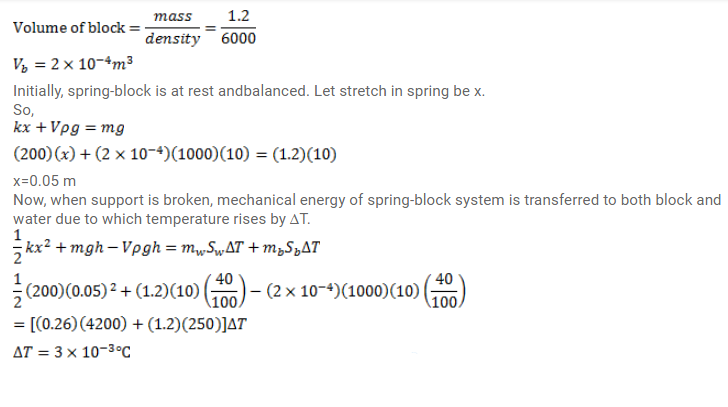# A metal block of density 6000 kg/m2 and mass 1.2 kgQuestion:

A metal block of density $6000 \mathrm{~kg} / \mathrm{m}^{3}$ and mass $1.2 \mathrm{~kg}$ is suspended through a spring of spring constant $200 \mathrm{~N} / \mathrm{m}$. The spring-block system is dipped in water kept in a vessel. The water has a mass of $260 \mathrm{~g}$ and the block is at a height $40 \mathrm{~cm}$ above the bottom of the vessel. If the support to the spring is broken, what will be the rise in the temperature of the water. Specific heat capacity of the block is $250 \mathrm{~J} / \mathrm{kg}-\mathrm{K}$ and that of water is $4200 \mathrm{~J} / \mathrm{kg}-\mathrm{K}$. Heat capacities of the vessel and the spring is negligible.

Solution: